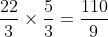## Example Questions

### Example Question #1 : Complex Fractions

Which of the following is equal to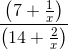?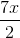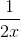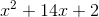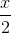Explanation:

First we must take the numerator of our whole problem. There is a fraction in the numerator withas the denominator. Therefore, we multiply the numerator of our whole problem by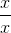, giving us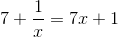.

Now we look at the denominator of the whole problem, and we see that there is another fraction present withas a denominator. So now, we multiply the denominator by, giving us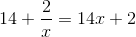.

Our fraction should now read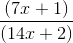. Now, we can factor our denominator, making the fraction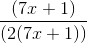.

Finally, we cancel out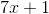from the top and the bottom, giving us.

### Example Question #2 : Complex Fractions

Simplify: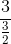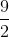Explanation:

Rewriteinto the following form: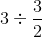Change the division sign to a multiplication sign by flipping the 2nd term and simplify.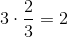### Example Question #1 : Complex Fractions

Evaluate: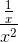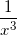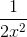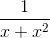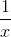Explanation:

The expressioncan be rewritten as: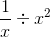Change the division sign to a multiplication sign and take the reciprocal of the second term.  Evaluate.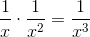### Example Question #4 : Complex Fractions

Simplify: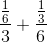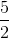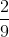Explanation:

The expressioncan be simplified as follows:

We can simplify each fraction by multiplying the numerator by the reciprocal of the denominator.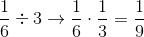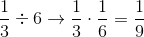From here we add our two new fractions together and simplify.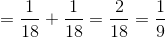### Example Question #5 : Complex Fractions

Simplify the following: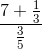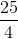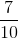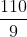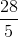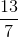Explanation:

Begin by simplifying your numerator. Thus, find the common denominator: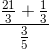Next, combine the fractions in the numerator: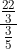Next, remember that to divide fractions, you multiply the numerator by the reciprocal of the denominator: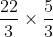Since nothing needs to be simplified, this is just: